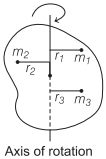# Moment of Inertia in Physics | Definition, Formula, Units – Rotational Motion

Moment of Inertia Definition:
The inertia of rotational motion is called moment of inertia. It is denoted by I.

We are giving a detailed and clear sheet on all Physics Notes that are very useful to understand the Basic Physics Concepts.

## Moment of Inertia in Physics | Definition, Formula, Units – Rotational Motion

1. Moment of inertia is the property of an object by virtue of which it opposes any change in its state of rotation about an axis.

2. The moment of inertia of a body about a given axis is equal to the sum of the products of the masses of its constituent particles and the square of their respective distances from the axis of rotation.Moment of inertia of a body,

$$I=m_{1} r_{1}^{2}+m_{2} r_{2}^{2}+m_{3} r_{3}^{2}+\ldots=\sum_{i=1}^{n} m_{i} r_{i}^{2}$$

Moment of Inertia Units:
SI unit is kg-m²

Moment of Inertia Dimensional Formula
Dimensional formula is [ML²].

The moment of inertia of a body depends upon:

• Position of the axis of rotation.
• Orientation of the axis of rotation.
• Shape and size of the body.
• Distribution of mass of the body about the axis of rotation.

The physical significance of the moment of inertia is same in rotational motion as the mass in linear motion.

Rotational Motion:
In this portion, we will learn about the rotational motion of the objects. A body moves completely in rotational motion when each particle of the body moves in a circle about a single line. When a force is applied on a body about an axis it causes a rotational motion. The force applied here is called the torque. The axis of the rotation usually goes through the body. Also, learn the two theorems such as parallel axes and perpendicular theorem explained with respect to rotational motion of objects.